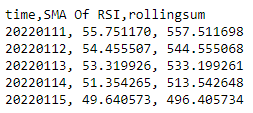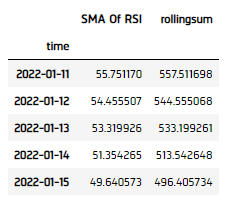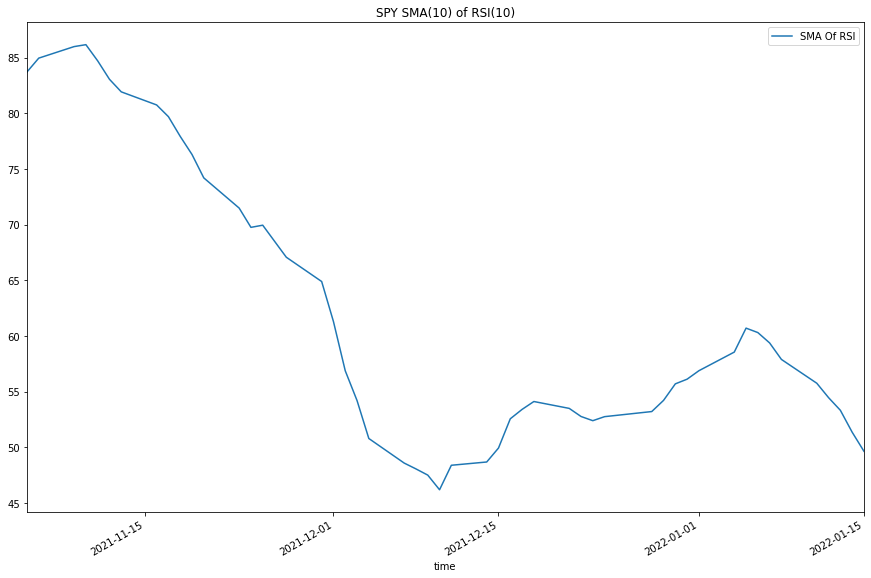# Indicators

## Combining Indicators

### Introduction

This page explains how to create, update, and visualize LEAN Composite indicators.

### Create Subscriptions

You need to subscribe to some market data in order to calculate indicator values.

var qb = new QuantBook();
var symbol = qb.AddEquity("SPY").Symbol;
qb = QuantBook()
symbol = qb.AddEquity("SPY").Symbol

### Create Indicator Timeseries

You need to subscribe to some market data and create a composite indicator in order to calculate a timeseries of indicator values. In this example, use a 10-period SimpleMovingAverage of a 10-period RelativeStrengthIndex indicator.

var rsi = new RelativeStrengthIndex(10);
var sma = new SimpleMovingAverage(10);
var smaOfRsi = IndicatorExtensions.Of(sma, rsi);
rsi = RelativeStrengthIndex(10)
sma = SimpleMovingAverage(10)
sma_of_rsi = IndicatorExtensions.Of(sma, rsi)

Follow these steps to create an indicator timeseries:

1. Get some historical data.
2. var history = qb.History(symbol, 70, Resolution.Daily);
history = qb.History[TradeBar](symbol, 70, Resolution.Daily)
3. Create a RollingWindow for each attribute of the indicator to hold their values.
4. In this example, save 50 data points.

var time = new RollingWindow<DateTime>(50);
var window = new Dictionary<string, RollingWindow<decimal>>();
window["SMA Of RSI"] = new RollingWindow<decimal>(50);
window["rollingsum"] = new RollingWindow<decimal>(50);

window = {}
window['time'] = RollingWindow[DateTime](50)
window["SMA Of RSI"] = RollingWindow[float](50)
window["rollingsum"] = RollingWindow[float](50)(50)

5. Attach a handler method to the indicator that updates the RollingWindow objects.
6. smaOfRsi.Updated += (sender, updated) =>
{
var indicator = (SimpleMovingAverage)sender;    // Use terminal indicator class.
};
def UpdateSmaOfRsiWindow(sender: object, updated: IndicatorDataPoint) -> None:
indicator = sender

sma_of_rsi.Updated += UpdateSmaOfRsiWindow

When the indicator receives new data, the preceding handler method adds the new IndicatorDataPoint values into the respective RollingWindow.

7. Iterate the historical market data to update the indicators and the RollingWindows.
8. foreach(var bar in history){
// Update the base indicators, the composite indicator will update automatically when the base indicator is updated.
rsi.Update(bar.EndTime, bar.Close);
}
for bar in history:
rsi.Update(bar.EndTime, bar.Close)
9. Display the data.
10. Console.WriteLine($"time,{string.Join(',', window.Select(kvp => kvp.Key))}"); foreach (var i in Enumerable.Range(0, 5).Reverse()) { var data = string.Join(", ", window.Select(kvp => Math.Round(kvp.Value[i],6))); Console.WriteLine($"{time[i]:yyyyMMdd}, {data}");
}11. Populate a DataFrame with the data in the RollingWindow objects.
12. sma_of_rsi_dataframe = pd.DataFrame(window).set_index('time')### Plot Indicators

Jupyter Notebooks don't currently support libraries to plot historical data, but we are working on adding the functionality. Until the functionality is added, use Python to plot composite indicators.

Follow these steps to plot the indicator values:

1. Select the columns/features to plot.
2. sma_of_rsi_plot = sma_of_rsi_dataframe[["SMA Of RSI"]]
3. Call the plot method.
4. sma_of_rsi_plot.plot(title="SPY SMA(10) of RSI(10)", figsize=(15, 10))
5. Show the plots.
6. plt.show()You can also see our Videos. You can also get in touch with us via Discord.